﻿ 与差分相关的整函数周期性问题

# 与差分相关的整函数周期性问题Periodicity of Entire Functions Concerning Difference

Abstract: This paper studied the periodicity of entire functions with its difference, and proved: Let f(z) be a transcendental entire function of finite order, and d be a Picard exceptional value of f(z). If f(z)Δcf(z) is a periodic function, then f(z) is also a periodic function.

1. 引言及主要结果是复平面上的整函数，定义其差分算子为

${\Delta }_{c}f\left(z\right)=f\left(z+c\right)-f\left(z\right)$, ${\Delta }_{c}^{n}f\left(z\right)={\Delta }_{c}\left({\Delta }_{c}^{n-1}f\left(z\right)\right)$.

2018年，王琼、扈培础  研究了杨重俊提出的如下猜想：

2019年，刘凯等人  研究了在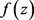有非零Picard例外值的情形，猜想1是正确的。

2c周期的周期函数。

2. 一些引理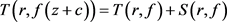.

$\underset{i=1}{\overset{n}{\sum }}N\left(r,\frac{1}{{f}_{j}}\right)+\left(n-1\right)\underset{i=1}{\overset{n}{\sum }}\stackrel{¯}{N}\left(r,{f}_{j}\right)<\left(\lambda +o\left(1\right)\right)T\left(r,{f}_{k}\right)$,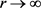, $r\notin E$,

${f}_{n}\left(z\right)\equiv 1$

3. 定理3的证明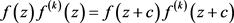.(1)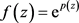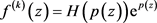代入(1)式，可得

$H\left(p\left(z\right)\right){\text{e}}^{2p\left(z\right)}=H\left(p\left(z+c\right)\right){\text{e}}^{2p\left(z+c\right)}$.

${\text{e}}^{2{\Delta }_{c}p\left(z\right)}=\frac{H\left(p\left(z\right)\right)}{H\left(p\left(z+c\right)\right)}$.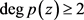时， $\mathrm{deg}{\Delta }_{c}p\left(z\right)=\mathrm{deg}p\left(z\right)-1\ge 1$.

$T\left(r,{\text{e}}^{{\Delta }_{c}p\left(z\right)}\right)=T\left(r,\frac{H\left(p\left(z\right)\right)}{H\left(p\left(z+c\right)\right)}\right)=S\left(r,{\text{e}}^{{\Delta }_{c}p\left(z\right)}\right)$,

4. 定理4的证明

$f\left(z\right){\Delta }_{c}f\left(z\right)=f\left(z+\eta \right){\Delta }_{c}f\left(z+\eta \right)$.(2)

$f\left(z\right)=d+{\text{e}}^{p\left(z\right)}$${\Delta }_{c}f\left(z\right)={\text{e}}^{p\left(z+c\right)}-{\text{e}}^{p\left(z\right)}$ 代入(2)式，

$c+\eta \ne 0$ 时，有

$d\left({\text{e}}^{{\Delta }_{c}p}-1-{\text{e}}^{{\Delta }_{c+\eta }p}+{\text{e}}^{{\Delta }_{\eta }p}\right){\text{e}}^{p}+\left({\text{e}}^{{\Delta }_{c}p}-1-{\text{e}}^{{\Delta }_{\eta }p+{\Delta }_{c+\eta }p}+{\text{e}}^{2{\Delta }_{\eta }p}\right){\text{e}}^{2p}\equiv 0$. (3)

$d\left({\text{e}}^{{\Delta }_{c}p}-1-{\text{e}}^{{\Delta }_{c+\eta }p}+{\text{e}}^{{\Delta }_{\eta }p}\right)\equiv 0$, (4)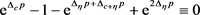. (5)

$T\left(r,{\text{e}}^{p}\right)=T\left(r,\frac{d\left({\text{e}}^{{\Delta }_{c}p}-1-{\text{e}}^{{\Delta }_{c+\eta }p}+{\text{e}}^{{\Delta }_{\eta }p}\right)}{{\text{e}}^{{\Delta }_{c}p}-1-{\text{e}}^{{\Delta }_{\eta }p+{\Delta }_{c+\eta }p}+{\text{e}}^{2{\Delta }_{\eta }p}}\right)=S\left(r,{\text{e}}^{p}\right)$.

$p\left(z\right)={a}_{n}{z}^{n}+{a}_{n-1}{z}^{n-1}+\cdots +{a}_{1}z+{a}_{0}$$n\ge 1$${a}_{n}\ne 0$，即 $\left({\text{e}}^{{a}_{1}c}-1\right)\left(1-{\text{e}}^{2{a}_{1}\eta }\right)\equiv 0$ 解方程，有 ${\text{e}}^{{a}_{1}c}=1$，或 ${\text{e}}^{2{a}_{1}\eta }=1$。即 $f\left(z\right)$ 是以c或 $2\eta$ 为周期的周期函数。进一步的，若 $d\ne 0$，结合(4)式，可得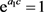，或 ${\text{e}}^{{a}_{1}\eta }=1$，即 $f\left(z\right)$ 是以c或 $\eta$ 为周期的周期函数。

$c+\eta =0$ 时，有

$d\left({\text{e}}^{{\Delta }_{c}p}-2+{\text{e}}^{{\Delta }_{\eta }p}\right){\text{e}}^{p}+\left({\text{e}}^{{\Delta }_{c}p}-1-{\text{e}}^{{\Delta }_{\eta }p}+{\text{e}}^{2{\Delta }_{\eta }p}\right){\text{e}}^{2p}\equiv 0$. (6)

 杨乐. 值分布及其新研究[M]. 北京: 科学出版社, 1982: 5-13.

 Yang, C.C. and Yi, H.X. (2003) Uniqueness Theory of Meromorphic Functions. Science Press, Beijing, 1-13.
https://doi.org/10.1007/978-94-017-3626-8_1

 Hayman, W.K. (1964) Mermorphic Functions. Clarendon Press, Oxford, 4-10.

 王琼, 扈培础. 关于整函数零点和周期性的研究[J]. 数学物理学报, 2018, 38(2): 209-204.

 Liu, K. and Yu, P.Y. (2019) A Note on the Periodicity of Entire Functions. Bulletin of the Australian Mathematical Society, 100, 290-296.
https://doi.org/10.1017/S0004972719000030

 Chiang, Y.M. and Feng, S.J. (2008) On the Nevanlinna Characteristic of f(z+η) and Difference Equations in the Complex Plane. The Ramanujan Journal, 16, 105-129.
https://doi.org/10.1007/s11139-007-9101-1

Top